NCERT Solutions for Class 6 Maths Chapter 10 Mensuration Ex 10.1 are part of NCERT Solutions for Class 6 Maths. Here we have given NCERT Solutions for Class 6 Maths Chapter 10 Mensuration Ex 10.1.

 Board CBSE Textbook NCERT Class Class 6 Subject Maths Chapter Chapter 10 Chapter Name Mensuration Exercise Ex 10.1 Number of Questions Solved 17 Category NCERT Solutions

## NCERT Solutions for Class 6 Maths Chapter 10 Mensuration Ex 10.1

Question 1.
Find the perimeter of each of the following figures: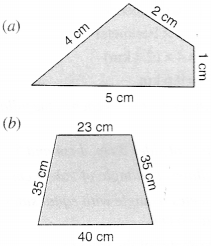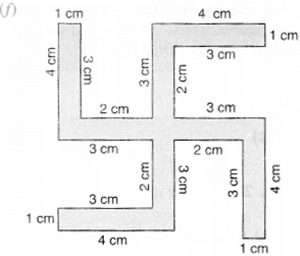Solution :
(a) Perimeter
= 5 cm + I cm + 2 cm + 4 cm
= 12 cm
(b) Perimeter
= 40 cm + 35 cm + 23 cm + 35 cm
= 133 cm
(c) Perimeter
= 15 cm + 15 cm + 15 cm+ 15 cm
= 60 cm
(d) Perimeter
= 4 cm + 4 cm + 4 cm + 4 cm + 4 cm
= 20 cm
(e) Perimeter
= 1 cm + 4 cm + 0.5 cm + 2.5 cm + 2.5 cm + 0.5 cm + 4 cm
= 15 cm
(f) Perimeter = 1 cm + 3 cm + 2 cm + 3 cm + 4 cm + 1 cm + 3 cm + 2 cm + 3 cm + 4 cm + 1 cm + 3 cm + 2 cm + 3 cm + 4 cm + 1 cm + 3 cm + 2 cm + 3 cm + 4 cm
= 52 cm

Question 2.
The lid of a rectangular box of sides 40 cm by 10 cm is sealed all around with tape. What is the length of the tape required?
Solution :
Length of the tape required
= Perimeter of the rectangular box = 2 × (Length + Breadth)
= 2 × (40 cm + 10 cm)
= 2 × (50 cm)
= 100 cm = l m.

Question 3.
A table-top measures 2 m 25 cm by l m 50 cm. What is the perimeter of the table-top?
Solution :
Perimeter of the table-top
= 2 × (Length + Breadth)
= 2 × (2 m 25 cm + 1 m 50 cm)
= 2 × (2.25 m+1.50 m)
= 2 × (3.75 m)
= 7.5 m

Question 4.
What is the length of the wooden strip required to frame a photograph of length and breadth 32 cm and 21 cm respectively?
Solution :
Length of the wooden strip required = 2 × (Length + Breadth)
= 2 × (32 cm + 21 cm)
= 2 × (53 cm)
= 106 cm = 1.06 m.

Question 5.
A rectangular piece of land measures 0.7 km by 0.5 km. Each side is to be fenced with 4 rows of wires. What is the length of the wire needed?
Solution :
Perimeter of the rectangle
= 2 × (Length + Breadth )
= 2 × (0.7 km + 0.5 km)
= 2 × (1.2km)
= 2.4 km
Length of the wire needed
= 4 × Perimeter of the rectangle = 4 × (2.4 km)
= 9.6 km.

Question 6.
Find the perimeter of each of the following shapes:
(a) A triangle of sides 3 cm, 4 cm, and 5 cm
(b) An equilateral triangle of side 9 cm
(c) An isosceles triangle with equal sides 8 cm each a mi third side 6 cm.
Solution :
(a) Perimeter of the triangle = 3 cm + 4 cm + 5 cm = 12 cm
(b) Perimeter of the equilateral triangle = 3 × Length of a side = 3 × (9 cm) = 27 cm
(c) Perimeter of the isosceles triangle = 8 cm + 8 cm + 6 cm = 22 cm.

Question 7.
Find the perimeter of a triangle with sides measuring 10 cm, 14 cm, and 15 cm.
Solution :
Perimeter of the triangle
= Sum of the lengths of its three sides
= 10 cm + 14 cm + 15 cm
= 39 cm.

Question 8.
Find the perimeter of a regular hexagon with each side measuring 8 m.
Solution :
Perimeter of the regular hexagon
= 6 × Length of a side
= 6 × (8m)
= 48 m.

Question 9.
Find the side of the square whose perimeter is 20 m.
Solution :
Perimeter of the square
= 4 × Length of a side
⇒ Length of one side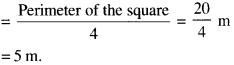Question 10.
The perimeter of a regular pentagon is 100 cm. How long is its every side?
Solution :
Perimeter of the regular pentagon = 5 × Length of a side
⇒ Length of one (each) side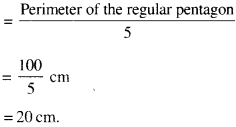Question 11.
A piece of string is 30 cm long. What will be the length of each side if the string is used to form:
(a) a square?
(b) an equilateral triangle?
(c) a regular hexagon?
Solution :
(a) Perimeter of the square = 4 × Length of a side
⇒ Length of a side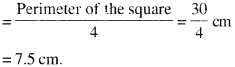(b) Perimeter of the equilateral triangle
= 3 × Length of a side
⇒ Length of a side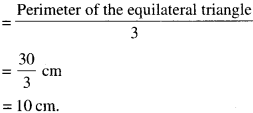(c)
Perimeter of the regular hexagon = 6 × Length of a side.
⇒ Length of a side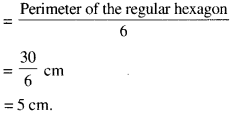Question 12.
Two sides of a triangle are 12 cm and 14 cm. The perimeter of the triangle is 36 cm. What is the third side?
Solution :
Perimeter of a triangle = Sum of the lengths of its three sides
⇒ 36 cm = 12 cm + 14 cm + Length of the third side
⇒ 36 cm = 26 cm + Length of the third side
⇒ Length of the third side = 36 cm – 26 cm = 10 cm.

Question 13.
Find the cost of fencing a square park of side 250 m at the rate of ₹ 20 per meter.
Solution :
Perimeter of the square park = 4 × Length of a side = 4 × (250m)
= 1000 m
∴ Cost of fencing the square park at the rate of?
20 per metre = ₹ 1000 × 20
= ₹ 20,000

Question 14.
Find the cost of fencing a rectangular park of length 175 m and breadth 125 m at the rate of? 12 per meter.
Solution :
Perimeter of the rectangular park = 2 × (Length + Breadth)
= 2 × (175m + 125 m)
= 2 × (300 m)
= 600 m
Cost of fencing the rectangular park at the rate of ?
12 per metre = ₹ 600 × 12
= ₹ 7200.

Question 15.
Sweety runs around a square park of side 75 m. Bulbul runs around a rectangular park with length 60 m and breadth 45 m. Who covers less distance?
Solution :
Perimeter of the square park = 4 × Length of a side = 4 × (75 m)
= 300 m
Perimeter of the rectangular park
= 2 × (Length + Breadth)
= 2 × (60 m + 45 m)
= 2 × (105 m)
= 210 m.
Since, the perimeter of the rectangular park is less than the perimeter of the square park, therefore. Bulbul covers less distance.

Question 16.
What is the perimeter of each of the following figures? What do you infer from the answers?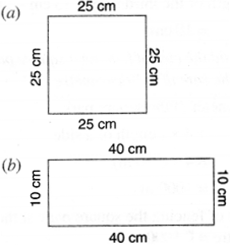Solution :
(a) Perimeter
= Sum of the lengths of all the sides
= 25 cm + 25 cm + 25 cm + 25 cm
= 100 cm.

(b) Perimeter
= Sum of the lengths of all the sides
= 40 cm + 10 cm + 40 cm + 10 cm
= 100 cm.

(c) Perimeter
= Sum of the lengths of all the sides
= 30 cm + 20 cm + 30 cm + 20 cm
= 100 cm.

(d) Perimeter
= Sum of the lengths of all the sides
= 30 cm + 40 cm + 30 cm
= 100 cm.
The inference from the answers. All the figures have the same perimeter.

Question 17.
Avneet buys 9 square paving slabs, each with a side of $$\frac { 1 }{ 2 }$$ m. He lays them in the form of a square.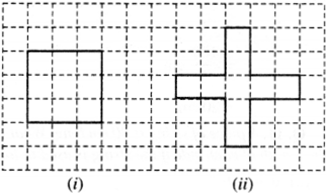(a) What is the perimeter of his arrangement (Fig. i)?
(b) Shari does not like his arrangement. She gets him to lay them out like a cross. What is the perimeter of her arrangement (Fig. ii)?
(c) Which has a greater perimeter?
(d) Avneet wonders if there is a way of getting an even greater perimeter. Can you find a way of doing this? (The paving slabs must meet along complete edges i.e. they cannot be broken.)
Solution :
(a) Perimeter of his arrangement = 4 × Length of one side(b) Perimeter of her arrangement = Sum of the lengths of all the sides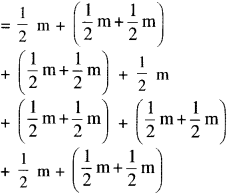(c) Cross has greater perimeter.
(d) Yes ! there is a way of getting an even greater perimeter. It is shown below: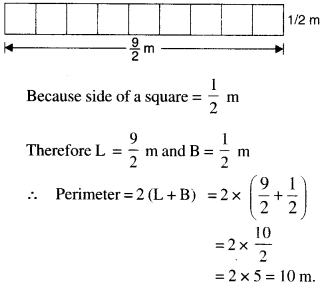We hope the NCERT Solutions for Class 6 Maths Chapter 10 Mensuration Ex 10.1 help you. If you have any query regarding NCERT Solutions for Class 6 Maths Chapter 10 Mensuration Ex 10.1, drop a comment below and we will get back to you at the earliest.